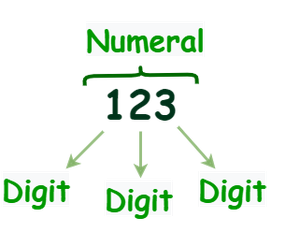# What is a digit in a number?

• Last Updated : 22 Sep, 2021

The number is an arithmetical representation of measurement and count that gives an idea. It can be represented in various ways. Say two or “2” otherwise denote by showing the two fingers. Without using numbers, one can not count anything such as money, date, time, etc. Sometimes numbers are used for measurement and sometimes for labeling.  Even without numbers, one can not calculate one’s marks too. There are various types of numbers such as whole number, even number, odd number, irrational number, rational number, etc.

What is a Numeral?

When one represents numbers in mathematical symbols or names, they are called numerals. It can be a symbol, figure, or group of figures or symbols. It is represented by numeric digits. Everyone is familiar with two different kinds of numerals Roman numeral and Hindu-Arabic numeral systems. Examples of Numerals: 1, 2,3 or One, Two, Three. The example of the roman numeral is I for 1, V for 5, VI for 6, X for 10, XIV for 14, and so on.

### What is a digit in a number?

Digit is an element of numerals. It comes from the Latin word finger. It is a single symbol that makes numbers. It can be any from 0 to 9. There are 10 digits available and all numerals made this combination of digits. Each digit represents an integer. People often tend to confuse between a number and a digit. A digit as very well known from the Hindu-Arabic numeral system contains 10 digits, they are 0, 1, 2, 3, 4, 5, 6, 7, 8, 9. While a number is defined as the string or combination of different/same digits.Digits 0 1 2 3 4 5 6 7 8 9

When numbers are only belonging to the first ten whole numbers, 0 to 9, they are known as 1 – digit numbers since only one digit is required. Numbers belonging from 10 to 99 are known as 2 – digit numbers as 2 digits are required to write down these numbers, similarly, numbers belonging from 100 to 999 are known as 3 – digit numbers, and so on.

### Conceptual Questions

Question 1: What is the smallest digit?

0 is the smallest digit.

Question 2: What is the biggest digit?

9 is the biggest digit.

Question 3: What is the highest number of 3 digits?

999 (Nine Hundred Ninty Nine) is the highest number of 3 digits.

Question 4: What is the lowest number of 4 digits?

1000 (One Thousand) is the lowest number of 4 digits.

Question 5: What will be the value of 27, if we interchange its digit place?